Technical Article

# Opposites Attract: A Review of Basic Magnetic Theories

June 08, 2015 by Editorial Team

## Electric machineries are based on the basic principles of electromechanical conversion, which use either the electrostatic or the electromagnetic principle. This technical article deals with the magnetic circuit theory for the conversion of one form of energy to another.

Electric machineries are based on the basic principles of electromechanical conversion. They use either the electrostatic or the electromagnetic principle. This technical article deals with the magnetic circuit theory for the conversion of one form of energy to another.

Beginner

#### Introduction

This technical article deals with the magnetic circuit theory for the conversion of one form of energy to another. A static device such as a transformer converts the electrical energy to electrical energy while rotating devices such as a DC machine, induction machine, or synchronous machine convert the mechanical or electrical energy into electrical or mechanical energy. Actuators, solenoids, and relays are also based on this conversion process. This conversion process happens in a magnetic material inside these machines. The magnetic material provides the high flux density which can provide high torque and high machine output per unit volume of the machines. This article is dedicated to the properties of these magnetic materials. We will see the basic methodology for the analysis of these machines by using their magnetic circuits.

#### A Review on Basic Magnetics

If we use a permanent magnet or let electric current flow through a coil, magnetic field is produced. The direction of magnetic field can be found out using the Right-hand rule which says that if the conductor is held in the right hand in such a way that the thumb indicates the direction of current, then the fingertips will indicate the direction of the magnetic field.

The basic laws related to magnetics are given below.

The EMF (or voltage) produced around a closed-loop coil is directly proportional to the rate of change of a magnetic field (time-variant) going through or out of that loop.

Thus,

$$EMF ∝ \frac{∂\Phi}{∂t}$$Figure 1. Magnetic Field (Varying with Time)

Lenz’s Law

According to this law, the direction of the electromagnetically-induced current is such that its magnetic field opposes direction of the original magnetic field that created the induced current. This is shown in the figure below.Figure 2. Direction for the Induced Current

As a result, the basic equation of Faraday's law of electromagnetic induction will have a negative sign.

Thus,

$$EMF=-\frac{∂\Phi}{∂t}$$

Ampere’s Law

This law is based on the discovery of the compass needles used for the detection of direction. We know that a current-carrying conductor produces a magnetic field. Lines of magnetic field form a closed path around the wire. The magnitude of the magnetic field density, B, is same on circular paths. B is directly proportional to the current and inversely proportional to the distance of a point on the closed path from the wire.

For a vector B and where dS is small element on the circular path,

$$\mathbf{B}\cdot d\boldsymbol{S} = B\,dS$$

Also,

$$B=\frac{µ_{0}I}{2πr}$$

where the value of B is constant around the closed path. Here, µ0  is the permeability of air.

Sum of the products for all such dS elements is given as,

$$\oint B\,dS=B\,\oint\,dS=\frac{µ_{0}I}{2πr}\,\oint dS$$

Consider a circular path, then

$$\oint dS = 2πr$$

We now have,

$$\oint B\,dS=µ_{0}I$$

The Ampere’s circuital law is that line integral of $$\mathbf{B} \cdot d\mathbf{S}$$ around any closed path is $$µ_{0}I$$. Here, $$I$$ is the total continuous current passing through any surface bounded by the closed path.

In terms of magnetic field intensity, this terms are reduced to:

$$\oint \mathbf{H}\cdot d\mathbf{l}=I_{Enclosed\,by\,path}$$

#### Parameters and Terminologies in Magnetics

Now, the basic terminologies are given for the construction of magnetic circuit. Later, we will see the process to form the magnetic equivalent circuit for a given machine.

Magnetic Flux Intensity (H)

If V is the potential of any point, then the electric current will produce a magnetic field of intensity H = -∇V.

The EMF in an electric circuit is analogous to the ampere-turns in a magnetic circuit. This gives the relationship between the current and field intensity.

The relationship between the current and field intensity is given by the Ampere’s circuital law mentioned.

$$\oint \mathbf{H}\cdot d\mathbf{l}=\sum{i}$$

Here,

$$\mathbf{H}$$ = magnetic field intensity at any point on the closed path of any shape

$$d\mathbf{I}$$= incremental length at the chosen point

Let the angle between the H and dI is Ө. Then

$$\oint H dL\,cosӨ=\sum{i}$$

But for a circular shape, the value of Ө = 0°. Thus, for a circular shape of radius r we have

$$H\, (2πr) = i_{T}$$

Where i= i+ i- i3 for the Figure 1. For a coil of N turns carrying i current in one conductor, i= Ni.Figure 3. Image to Illustrate Ampere Circuital Law

Magnetic Flux Density (B)

It is the flux per unit area. The flux in a magnetic circuit is analogous to the current in electric circuit. It is related to the flux density by the surface integral as shown by the following equation.

$$\Phi=\int_{S}\mathbf{B}\cdot d\mathbf{S}$$

Here, $$\Phi$$ is the flux expressed in Wb (Weber) measured for the surface area S.The unit of B is Wb/m2 or Tesla.

Reluctance

This is analogous to the electric resistance in the electric circuit but it is not necessarily a loss component in the magnetic circuit. The equation for the reluctance is given below which uses Ohm’s law and replaces the equivalent magnetic-circuit variables.

$$Reluctance,\,R=\frac{Magnetomotive\,Force}{Flux}$$

$$\Rightarrow R=\frac{NI}{\Phi} \text{ (At/Wb)}$$

Permeance (ᵱ)

It is the inverse of reluctance. This is used to portray the geometrical characteristics for magnetic field.

$$ᵱ=\frac{1}{R}$$

Leakage Flux

The entire magnetic flux through a magnet does not entirely pass through the low-reluctance path of the core; instead part of the flux also goes to the high-reluctance path of air or leaks out from the core.

As the current passes through the path of least resistance, the magnetic flux also has the ability of leaking out to the surrounding air. There is no magnetic insulator available to eliminate them, but magnetic shielding using DC or AC at low frequency can reduce it to some level. In the case of coupled circuits consisting of two or more coupled circuits having more than one winding, the leakage flux links to one coil  without interlinking others.

Fringing

This term is used to illustrate the deviations of the flux lines in an air gap of magnetic machine. Fringing is more significant  in air medium than to iron. Fringing increases the effective area of the gap. It is proportional to the length of the air gap.

Absolute and Relative Permeability (µ0, µr )

Permeability tells about the capability of the magnetic substance to favor the making of the magnetic field within itself. Absolute permeability is the ratio of the magnetic field density to the magnetic field intensity in a given medium given by

$$µ=\frac{B}{H}$$

Thus, absolute permeability of a material is given by the slope of the curve obtained between flux density and flux intensity for a particular value of the flux intensity.

The permeability changes with the change in flux intensity and change of the material as shown in the figure below. The different materials require the different values of current to establish a particular level of flux density.Figure 4. B-H Curve for Magnetic Materials

The flux density B increases linearly when the value of the  magnetic field intensity is low. However, as the value of the intensity is increased, flux density increases in a non-linear manner showing the effect of saturation. Hence, the reluctance of the magnetic path is based on the value of the flux density as shown in figure below.Figure 5. B-H Magnetization Curve

Relative permeability is a dimensionless quantity given by a ratio of a particular magnetic substance to that of a permeability of an air.

$$µ_{r}=\frac{µ}{µ_{0}}$$

Where µ0 is the permeability of air = 4 π X 10-7 Henry/meter.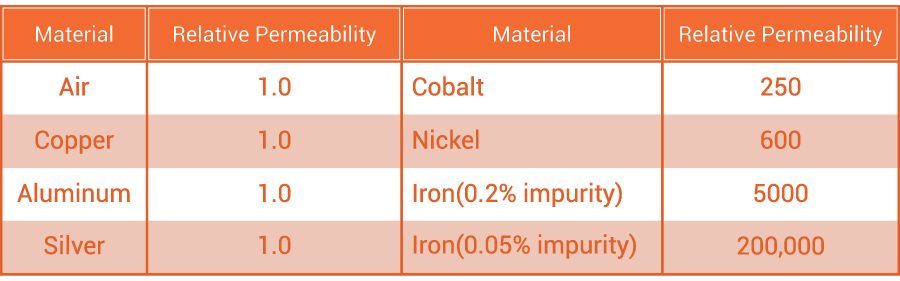Table 1. Relative Permeability of Few Materials

Inductance

A coil is usually wound on the magnetic core to generate the flux. This coil may be represented by the ideal element known as inductance represented by symbol L shown below. Inductance is the flux linkage of the coil per ampere of the current flowing through it.Figure 6. (a) Basic Magnetic Circuit (b) Equivalent Inductance for a Coil

$$L=\frac{N\Phi}{i}=\frac{N(BA)}{i}=Nµ\frac{HA}{i}=Nµ\frac{HA}{\frac{Hl}{N}}=\frac{{N}^{2}}{\frac{l}{µA}}=\frac{{N}^{2}}{R}$$

Note that inductance is proportional to the square of the number of turns on the coil.

Before starting the construction of the equivalent magnetic circuit, let us revise the basic analogy between the magnetic and electric circuit shown below.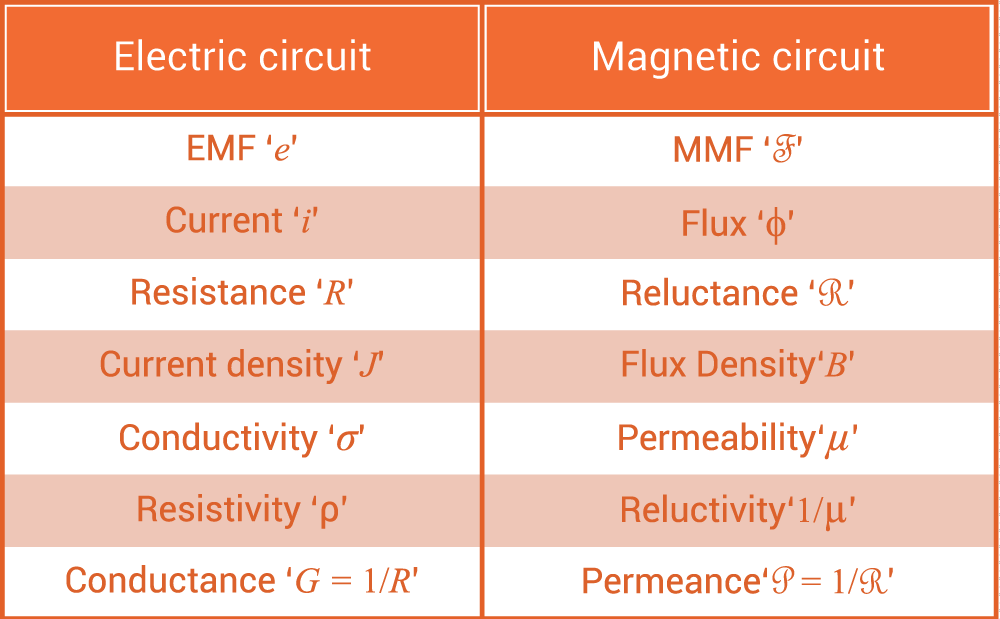Table 2. Electric and Magnetic Circuits Analogy

Equivalent Magnetic Circuit

The usefulness of the equivalent magnetic circuit is to find out the proper size of magnetic parts of an electric device during the design process, i.e. in finding out the parameters such as inductance and in finding out the air gap flux density for the calculation of power and torque.

The flux density in the core increases with the presence of ferromagnetic material or current-carrying coils. This in turn affects the inductance of the coil.

Although the magnetic field is a distributed parameter phenomenon, we can use the lumped parameter analysis for a definite class of magnetic material as done in the electric circuit analysis. However, the accuracy and precision for such analysis is less than the electric circuit analysis.

Consider a simple magnetic circuit having a ring-shaped magnetic core known as toroid as shown in figure below.Figure 7. A Toroid

The coil is wrapped around the entire circumference and is carrying the current i through a coil making N turns.

Let the leakage flux is nil as it is mostly confined within the core material.

From Ampere’s circuital law, we have,

$$\oint \mathbf{H}\cdot d\mathbf{l}=Ni$$

$$\Rightarrow H \cdot l=Ni=magnetomotive\,force = F \text{ (At)}$$

$$(l=2\,π\,r)$$

We know,

$$B = µ\, H \Rightarrow B=µ\frac{Ni}{l} \text{ (Tesla)}$$

As there is no leakage flux, the flux covering the cross section of the toroid is given by

$$\Phi=\oint \mathbf{B}\cdot d\mathbf{A}=B\,A\Rightarrow µ\frac{Ni}{l}A=\frac{Ni}{\frac{l}{µA}}=\frac{Ni}{R}$$

Here, $$\Re$$ is the reluctance of magnetic path given by

$$R=\frac{l}{µA}=\frac{1}{ᵱ}$$

The magnetic equivalent circuit for a toroid can be represented as shown below, which is basically derived from the analogous electric circuit. In this example, we have considered the circular core, but it can be of another form also such as in rectangular form.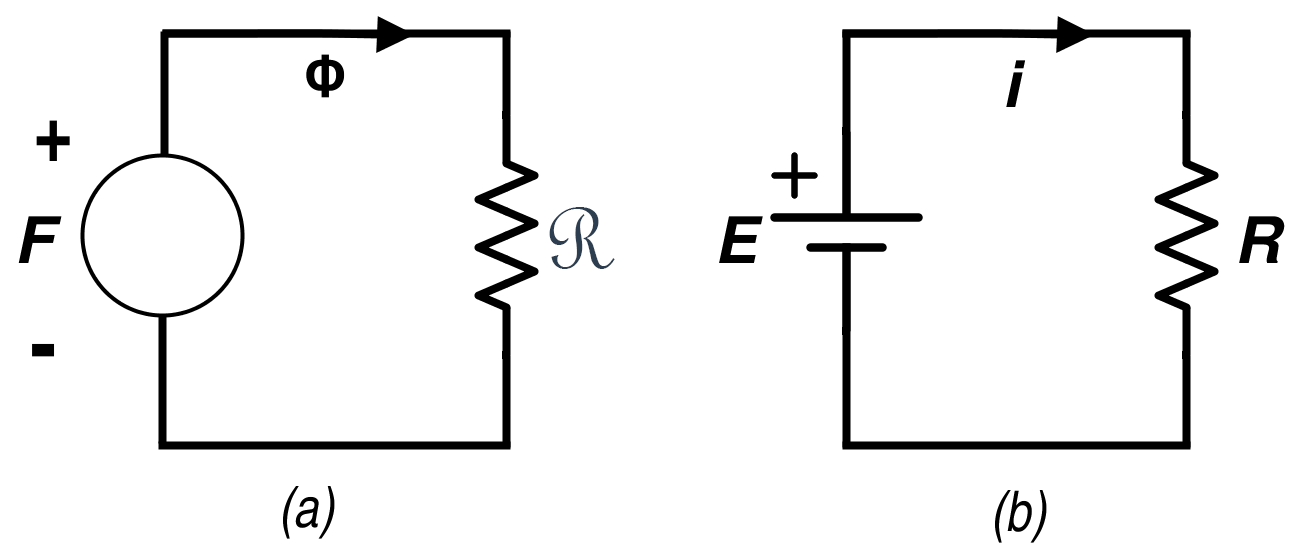Figure 8. (a) Equivalent Magnetic Circuit for a Toroid (b) Equivalent Electric circuit

Equivalent Magnetic Circuit for a Core with Multiple Excitations

Consider the magnetic device shown in Fig. 9 consisting of three coils for excitation carrying the currents  i1, i2, i3.

Consider the mean length of this magnetic circuit is L. The coils have turns ratio N1, N2, N3.The first two coils are producing the magnetic fluxes $$\Phi_{1}$$, $$\Phi_{2}$$ in the same direction while the direction of the current in the third coil is in such a way that it produces the flux $$\Phi_{3}$$ in the opposite direction.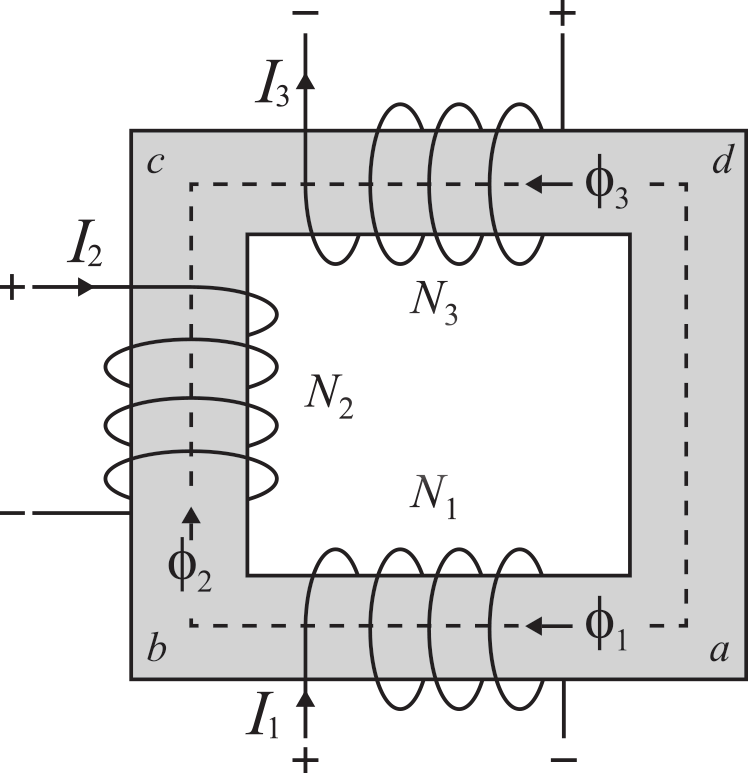Figure 9. Magnetic Device having Multiple Excitations

According to Ampere’s circuital law, integral of the magnetic field intensity around any closed path is equal to the total algebraic sum of the electric current in that path.

$$\int_{a}^{b}H\,dl=Ni=F=R\Phi=\frac{l}{µA}\Phi$$

Magnetic flux through surface S is given by

$$\Phi=\int_{S}\mathbf{B} \cdot d\mathbf{S}$$

If there is no saturation, then the value of the magnetic field intensity H varies linearly with the change in the  magnetic field density B.

The net magnetomotive force (mmf) is given by

$$\oint H\,dl=\int_{a}^{b}H_{K}dl\,+\,\int_{b}^{c}H_{K}dl\,+\,\int_{c}^{d}H_{K}dl\,+\,\int_{d}^{a}H_{K}dl$$

$$\Rightarrow \oint H\,dl=H_{K}L_{ab}\,+\,H_{K}L_{bc}\,+\,H_{K}L_{cd}\,+\,H_{K}L_{da}=H_{K}L=\Phi R=F=N_{1}I_{1}\,+\,N_{2}I_{2}\,-\,N_{3}I_{3}$$

Thus, the algebraic sum of magnetic potential around the closed path is zero. (Analogous of the KVL in electric circuit)

Thus, the magnetic circuit representation will be: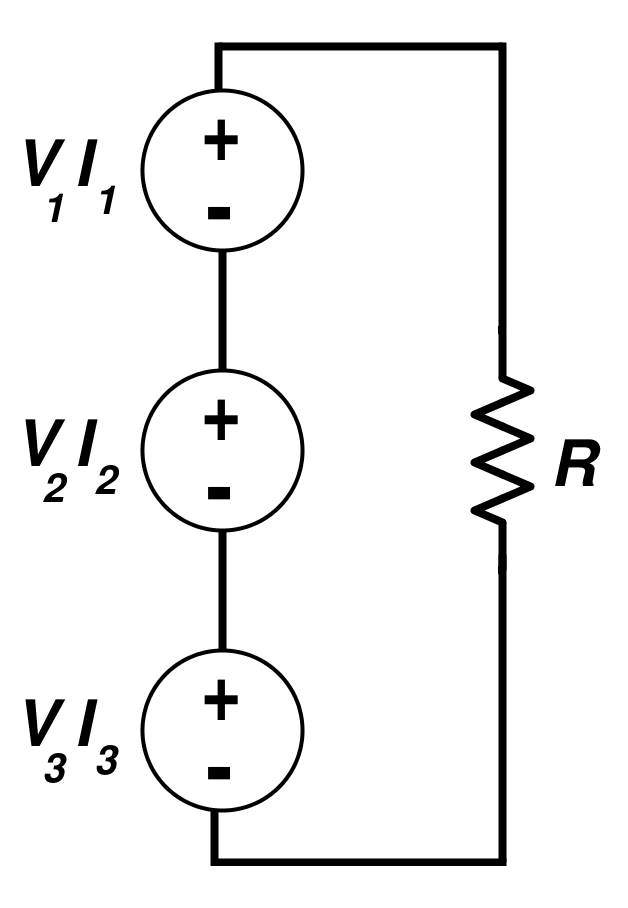Figure 10. Equivalent Circuit Representation for the Multiple Excitation System

Equivalent Magnetic Circuit with an Air Gap

In an electric machine, input and output of a magnetic system is isolated from the air gap. Practically, the same flux is required in the magnetic core and the air gap. Thus, an air will require more mmf than the core due to high reluctance. If the value of flux density is high, the core will exhibit saturation. However, the air gap will not get saturated as B-H curve for the air medium is linear (µ is constant for an air medium).

Consider the magnetic structure with a single coil and an air gap having mean length L as shown below.Figure 11. Magnetic Core having an Air Gap

$$mmf,\;F = Ni$$

Let the core have the reluctance RC and air gap have the reluctance Rg which is given by the following equations as follows:

$$R_{C}=\frac{l_{c}}{µ_{c}A_{C}}$$  and  $$R_{g}=\frac{l_{g}}{µ_{0}A_{g}}$$

$$Flux,\, \Phi=\frac{Ni}{R_{C}+R_{g}}$$

$$mmf, \;Ni = H_{C}\,l_{c}+H_{g}\,l_{g}$$

Consider that the air gap is of small size (usual). Thus, fringing effect can be ignored. Also, assume there is no saturation. Then,

$$Ni = H_{C}\,l_{c} + H_{g}\,l_{g} = H_{K}L$$

and

$$A_{g} = A_{C}$$

The value of flux density will be same both in its core and the air gap given by the ratio of flux to the cross-section area of the core. The equivalent circuit in this case is shown below.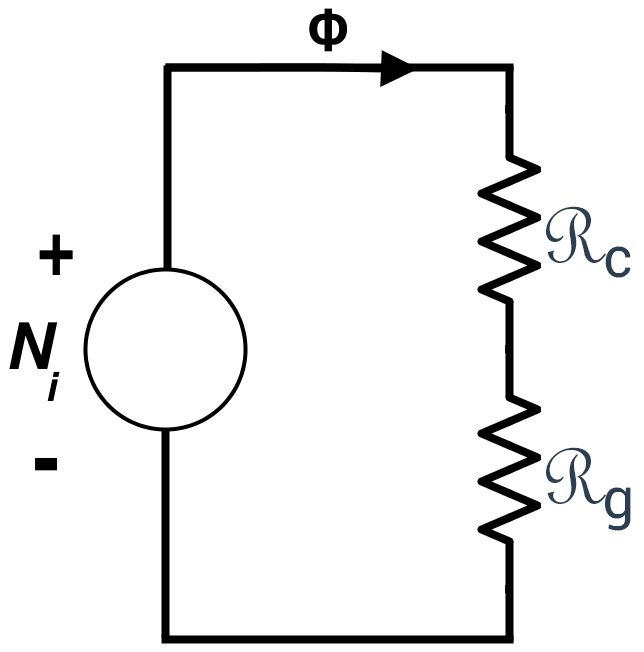Figure 12. Equivalent Circuit for Magnetic Core with an Air Gap